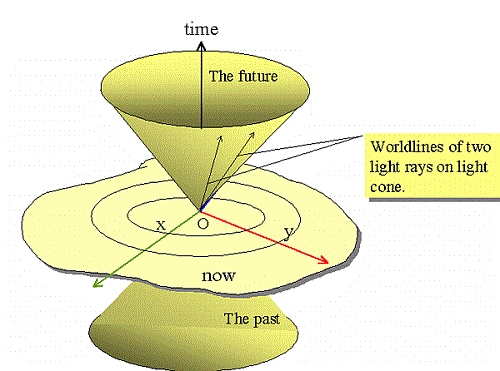What Is Spacetime?

This section provides a quick introduction of speed, which is a mathematical model used to describe our universe in frame of references that combines space and time together.

What Is Spacetime? Spacetime is a mathematical model used to describe our universe in frame of references that combines space and time together. A coordinate system with 4 dimensions can be associated with a spacetime frame,

Everything in our universe is made up by a set of events. Each event can be mapped to a point in a specific coordinate system associated with a specific spacetime frame of reference.

For example, we can define a spacetime frame of reference and coordinate system in spacetime as describe below:

• Set spacetime frame of reference to be fixed on yourself.
• Set the t-axis to represent the time and pointing to the future.
• Set the origin of time be the moment you are reading this book.
• Set the x, y, and z axes to represent space around you.
• Set the origin of space to be the location where are reading this book.

With this frame of reference and coordinate system defined for spacetime, we can describe some events with coordinate numbers now:

• (t,x,y,z) = (0,0,0,0) - The event of you reading this book.
• (t,x,y,z) = (-30y,0,0,0) - The event of you born (assuming you are 30 years old).
• (t,x,y,z) = (0,10m,0,0) - Some event that happens 10 meters away from you while you are reading this book.
• (t,x,y,z) = (-500s,149597870km,0,0) - The light left Sun 500 seconds ago and you observe it now.

Since 4-dimensional coodinate systems are very difficult to draw on paper, we can only try to illustrate spacetime by removing the z-axis as shown in the picture below:Spacetime with a Frame of Reference and Coordinates

Last update: 2014.